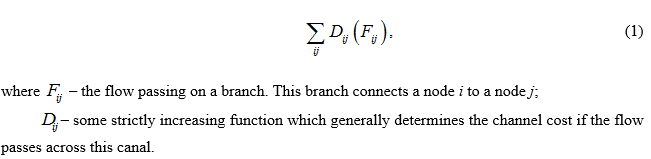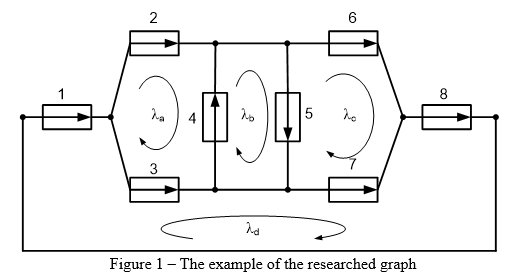Евразийский
научный
журнал# Comparative analysis of methods to solve the optimum routing taskПоделитесь статьей с друзьями:
Автор(ы): Пудалев Тимофей Олегович, Пронькин Леонид Александрович, Сутько Татьяна Александровна, Явношанов Дмитрий Александрович
Рубрика: Технические науки
Журнал: «Евразийский Научный Журнал №12 2015»  (декабрь 2015)
Количество просмотров статьи: 1444
Comparative analysis of methods to solve the optimum routing task

Pronkin Leonid,
Pudalev Timofey,
Sutko Tatyana,
Yavnoshanov Dmitriy
students of Siberian Federal University,
Krasnoyarsk, Russia,
E-mail: pudalev@gmail.com

Annotation

In this article, the method to optimize the traffic with the application of a planimetric model of a network is suggested. It allows reducing volume of calculations the criterion function, it also helps to reduce number of linearly independent variables of the criterion function, that will allow to reduce time of calculation of a minimum value.

Keywords: optimum routing, planimetric model, target function, algorithms.

For the graph like set structure a network in a kind of a count. It is necessary to define all possible focused ways between each pair source-addressee. The basic mathematical model for the decision of the problem of optimum routing was offered in [1, 2]. The complexity of such problem according to  for the generalized algorithm of Danzig or Floyd is O (2V3). As a result of this algorithm will be found kmn routes, where kmn means that k routes there are exist between n and m knots. These routes must not have loops. Based on the found routes, it is possible to get both a mathematical model of streams distribution, and the target function like:Flow is the amount of flows of kmn of the nodes n and m passing through this branch for each couple. Thus, if we have S possible couples between sources and receivers, the quantity of variables will be equal in target function to the work S kmn. As the full flow between couple of nodes n and m is usually known, one of k of flows can be expressed through other k-1 of flows. Then the total quantity linearly of independent variables will be equal in target function to S (kmn-1).

It is possible to offer other approach based on the tensor analysis of distributed systems of information processing. In the offered method, other approach to receiving a mathematical model of distributed systems  in which all flows of branches express through system of fundamental or linear and independent cycles of a graph is used. If to apply such model of the description of traffic distribution on a network, the number of operations on search of variables for target function will be reduced. It occurs because the search algorithm of fundamental cycles is based on the search algorithm in width or algorithm of creation of a spanning tree which complexity is described by the O(V)  function. Quantity of cycles to equally cyclomatic number of the graph r. Thus, it is necessary to mark that r≤kmn, therefore, number of independent variables for target function will be less that will lead to faster search of a minimum of target function.

For an example, we will find optimum allocation of traffic according to a graph with the following topology, a figure 1. In branches of a graph, there is a queuing system (QS) as a mathematical model of service of information units.In this example, the flow is generated in a branch 1 and ends in a branch 8. Closing of a branch 1 with a branch 8 is necessary for support of saving circulation of a flow . The system of linear and independent planimetric intensivnost allows defining single-digit loading in any branch through the following system of equations:Thus, the offered method allows to define target function with smaller costs of computation and to find its minimum. Besides complexity of algorithm of receiving target function and quantity of independent variables in target function grow linearly with growth of quantity of branches, in difference from the compared algorithms where complexity of computation is described by degree dependence.

Bibliography

1. Bertsekas D., Gallager R., Data networks, – Prentice-Hall International, Inc, – 544 p.
2. Vishnevsky V., Theoretical bases of design of computer networks – 512 p.
3. Maynika E., Algorithms of optimization on networks and graphs – 323 p.
4. Gaipov K., Zalenskaya M., Application of a tensor method of dual networks for the analysis of distributed systems of information processing.//The modern problems of science and education. – 2013. – No. 4. Access mode: http://www .science-education.ru/110-9766
5. Phillips D., García-Diaz A., Methods of the analysis of networks – 496 p.